# Home Work Set # 2, Physics 217, Due: September 19, 2001

### Problem 1

Check the fundamental theorem for gradients, using the function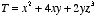and the points a = (0, 0, 0) and b = (1, 1, 1), and the following three paths (see Figure 1).
a) (0, 0, 0) → (1, 0, 0) → (1, 1, 0) → (1, 1, 1)
b) (0, 0, 0) → (0, 0, 1) → (0, 1, 1) → (1, 1, 1)
c) The parabolic path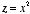;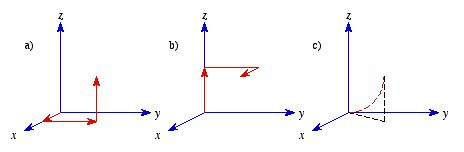Figure 1. Problem 1.

### Problem 2

Evaluate the following integrals:
a)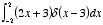b)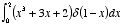c)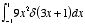d)### Problem 3

One consequence of the fundamental theorem for curls is that the surface integral of the curl of a vector function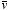depends only on the boundary line, not on the particular surface used. Consider the following vector function: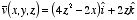a) Calculate the line integral ofalong the boundary of the square shown in Figure 2a.
b) Calculate the surface integral of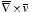over the surface of the square shown in Figure 2a.
c) Calculate the surface integral ofby integrating over the five sides of the cube shown in Figure 2b.
d) Compare the result of part a) with the result of part b) and c). What do you conclude?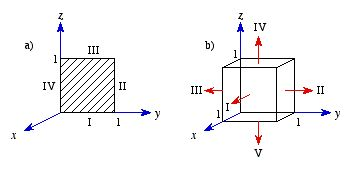Figure 2. Problem 3.

### Problem 4

a) Show that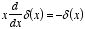Hint: Use integration by parts.
b) Let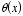be the "step function":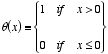Show that.

### Problem 5

a) Let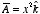and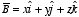. Calculate the divergence and curl of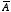and. Which one can be written as the gradient of a scalar? Find a scalar potential that does the job. Which one can be written as the curl of a vector function? Find a suitable vector potential.
b) Show that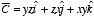can be written both as the gradient of a scalar and as the curl of a vector. Find scalar and vector potentials for this function.

### Problem 6

a) Compute the divergence of the function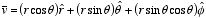b) Check the divergence theorem for this function, using as you volume the inverted hemisphere of radius R, resting on the x-y plane and centered at the origin (see Figure 3).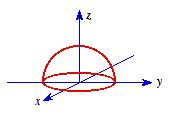Figure 3. Problem 6.

### Problem 7

Compute the line integral of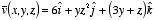along the triangular path shown in Figure 4. Check your answer using Stokes' theorem.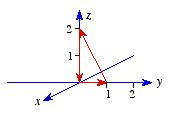Figure 4. Problem 6.